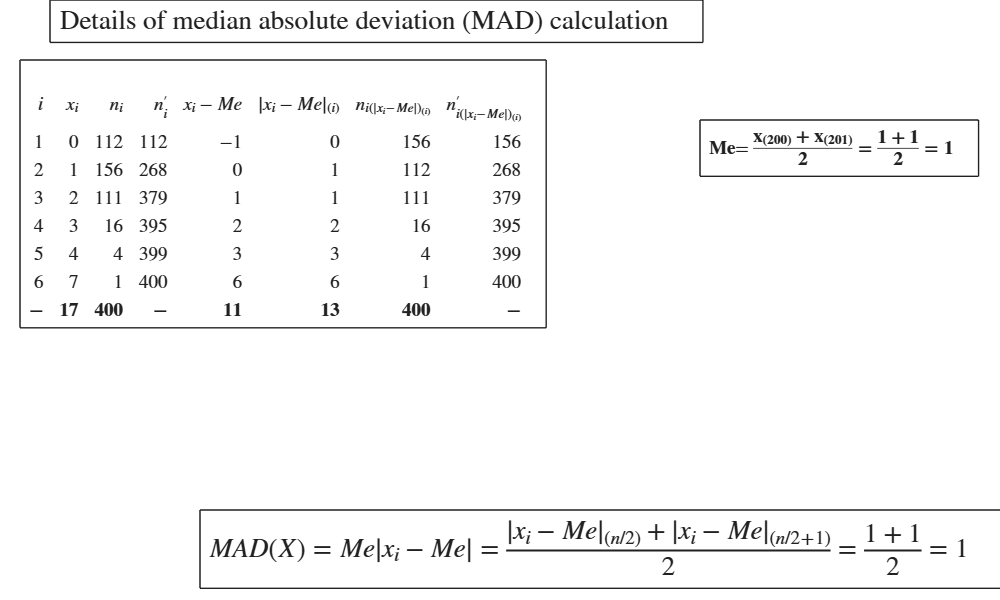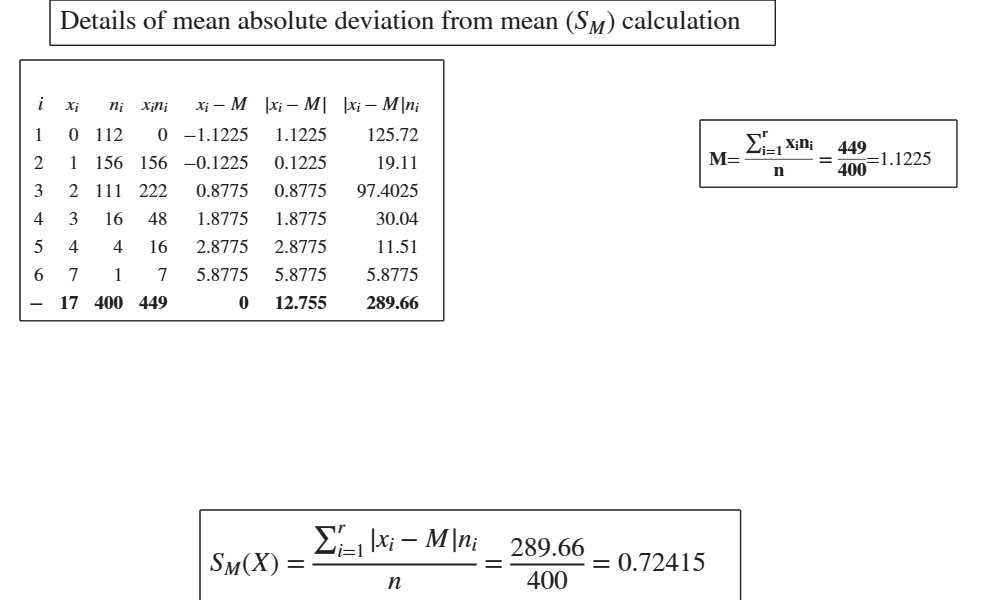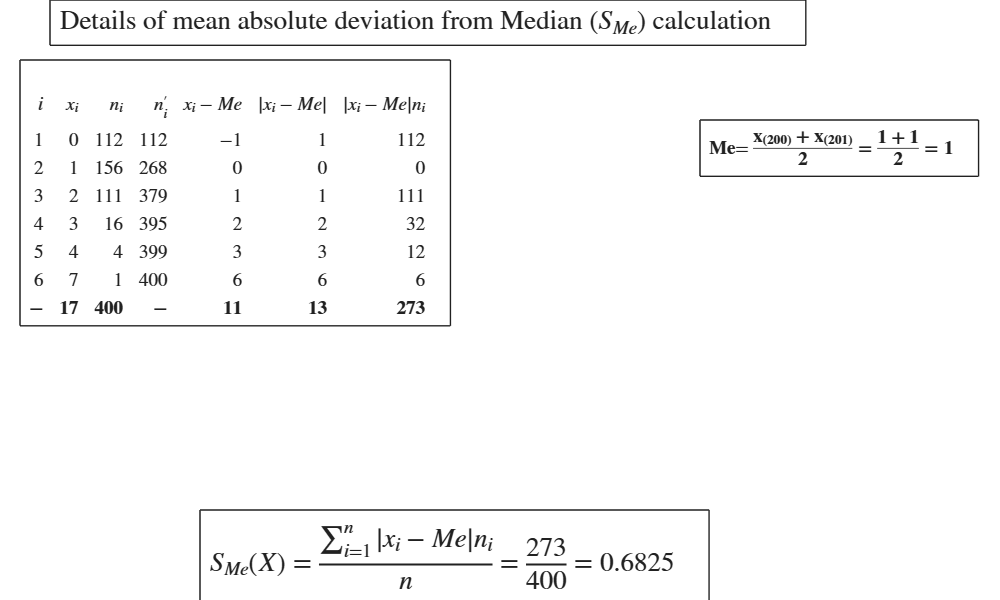Documentation

GUImad shows the necessary calculations to obtain MAD, S_M or S_Me in a GUI.

Description

This routine shows all the intermediate necessary steps to compute the three following variability indexes:

$MAD =Me(|x_{i} - Me|n_i).$ $S_{M} = \frac{\sum_{i=1}^{r}|x_{i}-M| n_i}{n}$ $S_{Me} = \frac{\sum_{i=1}^r |x_i-Me|n_i}{n}.$

out =GUImad(x, flag) Example of calculation of SMe.

out =GUImad(x, flag, w) Example of calculation of SM.

Examples

expand all

MAD = median absolute deviation from median.

x=[98 105 85 110 102];

Example of calculation of SMe.

SMe= mean absolute deviation from the median.

x=[98 105 85 110 102];

Example of calculation of SM.

SM= mean absolute deviation from mean.

x=[98 105 85 110 102];

Related Examples

expand allMAD in a frequency distribution.

MAD = median absolute deviation from median.

% Frequency distribution of the number of children in a sample of 400
% families. (See page 29 of [CMR]).
X=[0	112
1	156
2	111
3	16
4	4
7	1];
x=X(:,1);
freq=X(:,2);
flag=1;SM in a frequency distribution.

SM= mean absolute deviation from mean.

% Frequency distribution of the number of children in a sample of 400
% families. (See page 29 of [CMR]).
X=[0	112
1	156
2	111
3	16
4	4
7	1];
x=X(:,1);
freq=X(:,2);
flag=0;SMe in a frequency distribution.

SMe= median absolute deviation from mean.

% Frequency distribution of the number of children in a sample of 400 families. (See page 29 of [CMR]).
X=[0	112
1	156
2	111
3	16
4	4
7	1];
x=X(:,1);
freq=X(:,2);
flag=2;Input Arguments

x — vector of numeric data. Vector.

Vector containing strictly numerical data.

Data Types: double

flag — median or mean absolute deviation from median or mean absolute deviation from mean. Scalar.

If flag=1 (default), MAD is based on medians, i.e. median(abs(x-median(x)).

elseif flag=0, $S_M$ is computed (mean absolute deviation) i.e. mean(abs(x-mean(x)).

elseif flag=2, $S_{Me}$ is computed (mean absolute deviation from median) i.e. mean(abs(x-median(x)).

Example: 1

Data Types: double

w — weights. Vector.

Vector of the same length of x containing the weights assigned to each observation.

Example: 1:10

Data Types: double

Output Arguments

out —detailed output to compute the index. Table

Table with n+1 rows (where n is the length of x) containing what is shown in the GUI. Last row contains the totals.

Cerioli, A., Milioli, M.A., Riani, M. (2016), "Esercizi di statistica (Quinta edizione)". [CMR]